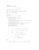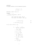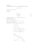# Exercise sheet 3 pdfLoading previews...differential equations and Laplace transformsdifferential equations and Laplace transformssimultaneous equations and Laplace transformspartial differential equations and Laplace transforms
4 files in this resource

## Exercise sheet 3 pdf

Exercises and solutions in PDFView Item